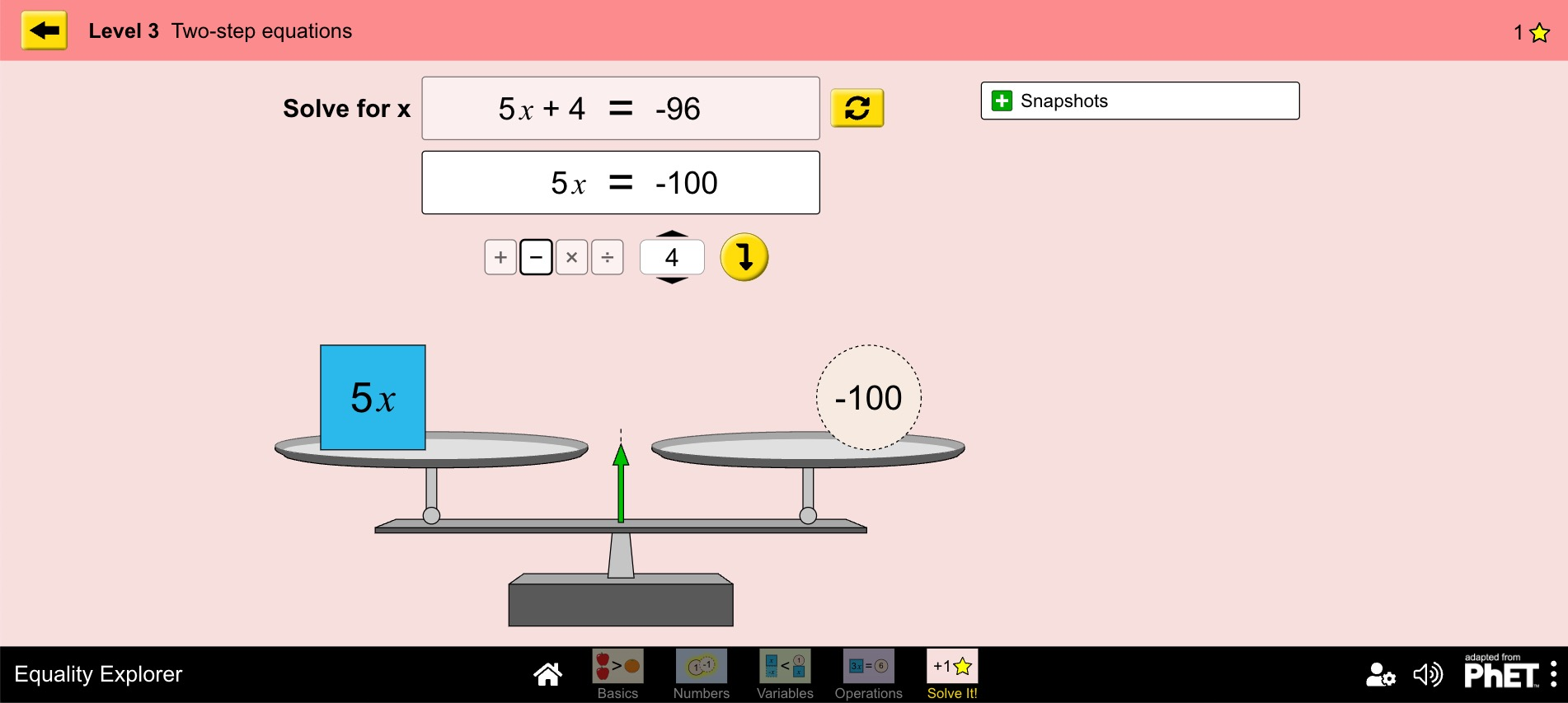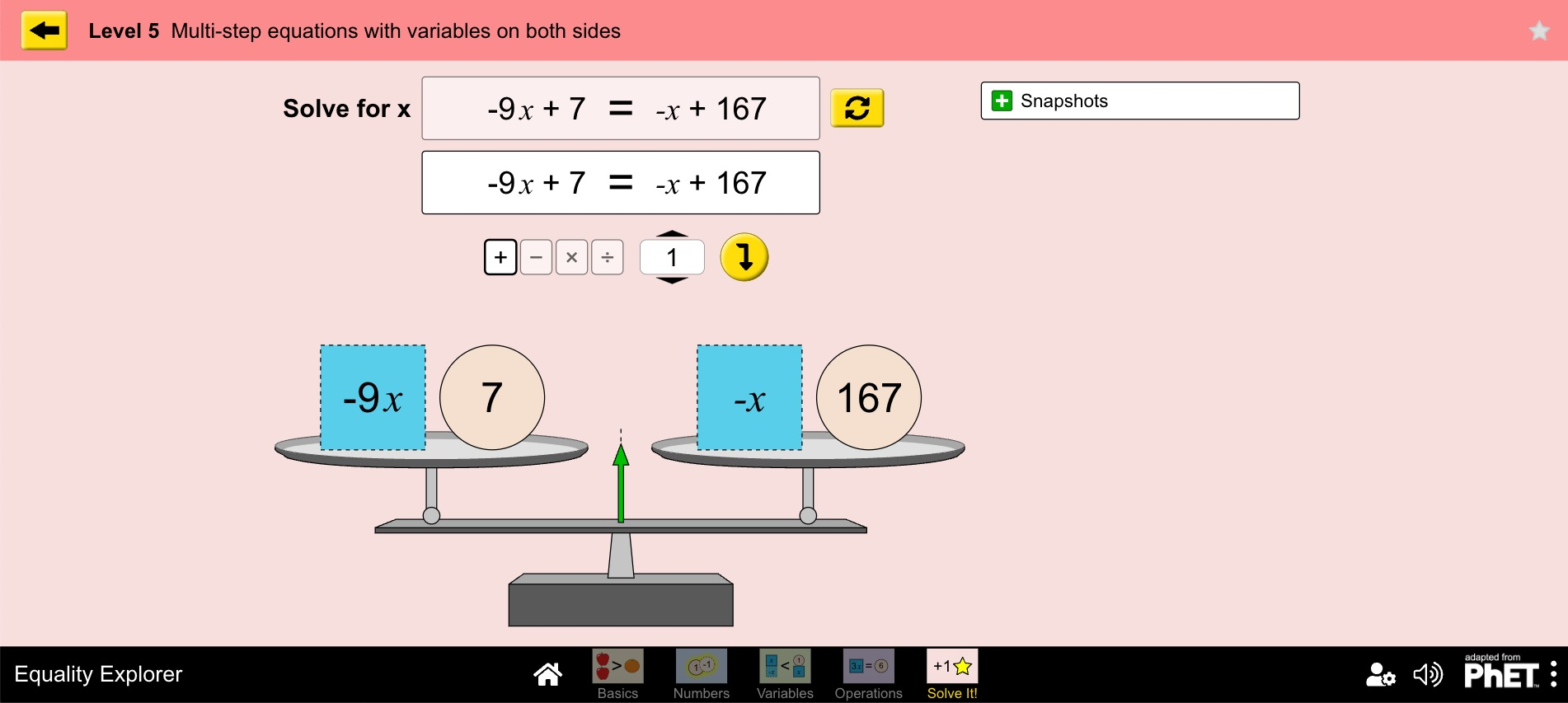MM Practice
×
Multiplication
Division
Place Value
Fractions & Decimals
Measurement
Statistics & Probability
Pre-Algebra
Money
Kindergarten

# Equality ExplorerModel and solve equations & inequalities on a pan balance (6th-8th grade)

In this activity, you can explore equality vs. inequality of mathematical expressions, and model and solve equations and inequalities, all with using a pan balance (scales).

There are five sections:

1. In the first section, you can explore equality of shapes, fruits, numbers, and more, using a simple pan balance. You can figure out, for example, how much a square weighs in terms of numbers, or the quantity of cats that equals another quantity of turtles in weight.
2. The second section has positive and negative counters and a pan balance. You can make an equation (where the balance is balanced) or an inequality (where the balance is not balanced), and explore what happens if you add negative counters to both sides.
3. In the third section you can model both inequalities and equations on a pan balance using the variable x and counters. Once you've built the equation or inequality, use the LOCK icon to add the same quantity to both sides at once. You cannot, however, divide both sides by some number (you can in the next section).
4. In the fourth section, you can build an equation or inequality on the pan balance, and apply the same operation to both sides.
5. In the last, "Solve It" section, you get to actually practice solving equations that are given to you. There are five levels:
Level 1: One-step equations
Level 2: One-step equations with negative coefficients
Level 3: Two-step equations
Level 4: Multi-step equations with fractions
Level 5: Variable on both sides

All equations/inequalities are linear, thus the activities are suitable for middle school (grades 6-8) and algebra 1.

Credit: This activity is created by PhET

Screenshots from the game and activity:Allow my comment to be posted on this site